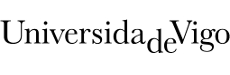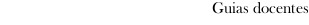Guia docente 2019_20Escuela de Ingeniería Industrial
 Degree in Mechanical EngineeringSubjectsPhysics: Physics IContents
 Topic Sub-topic 1.- UNITS, PHYSICAL AMOUNTS AND VECTORS 1.1.- The nature of Physics. 1.2.- Consistency and conversions of units. 1.3.- Uncertainty and significant figures. 1.4.- Estimates and orders of magnitude. 1.5.- Vectors and sum of vectors. 1.6.- Vector components. 1.7.- Unitary vectors. 1.8.- Vector products. 1.9.- Sliding Vectors 2.- CINEMATIC OF THE POINT 2.1.- Vectors of position, speed and acceleration. Half and instantaneous values 2.2.- Vectors angular speed and angular acceleration. Half and instantaneous values. 2.3.- Relation between linear cinematic magnitudes and angular 2.4.- Intrinsic components. 2.5.- Study of simple movements: *mov. Rectilinear, *mov. Circulate, shot *oblicuo 2.6.- Expressions of cinematic magnitudes in coordinates *cartesianas and polar 3.- LAWS OF THE MOVEMENT OF NEWTON 3.1.- Strength and interactions. 3.2.- First law of Newton. Systems of inertial and non inertial references 3.3.- Second law of Newton. 3.4.- Mass and weight. 3.5.- Third law of Newton. 3.6.- Quantity of movement. Mechanical impulse. Angular moment. 3.7.- Strengths of contact: active, of *ligadura. 4.- WORK AND KINETIC ENERGY 4.1.- Work realized by a Force. Power. 4.2.- Kinetic Energy. 4.3.- Conservative Forces 4.4.- Elastic potential energy. 4.5.- Potential energy in the gravitatory field. 4.6.- Mechanical energy. 4.7.- Strength and potential energy. 4.8.- Principle of conservation of the mechanical energy. 5.- KINEMATICS OF SYSTEM OF POINTS 5.1.- Points system. 5.2.- Rigid solid. 5.3.- Translation movement. 5.4.- Movement of rotation around a fixed axis. 5.5.- General movement. 5.6.- Instant center of rotation. 5.7.- Rolling motion. 5.8.- Relative movement. 6.- DYNAMICS OF THE SYSTEMS OF PARTICLES 6.1.- Systems of particles. Inner and exterior strengths. 6.2.- Center of masses of the system. Movement of the c.o.m. 6.3.- Equations of the movement of a system of particles. 6.4.- Linear moment. Theorem Of conservation. 6.5.- Angular moment of a system of particles. Theorem Of conservation. 6.6.- Work and power. 6.7.- Potential energy and kinetics of a system of particles. 6.8.- Theorem Of the energy of a system of particles. 6.9.- Crashes. 7.- DYNAMICS OF THE RIGID SOLID 7.1.- Rotation of a rigid solid around a fixed axis. 7.2.- Moments and products of inertia. 7.3.- Calculation of moments of inertia. 7.4.- Steiner's theorem. 7.5.- Moment of a force and pair of forces. 7.6.- Equations of the general movement of the rigid solid. 7.7.- Kinetic energy in the general movement of the rigid solid. 7.8.-Work in the general movement of the rigid solid. 7.9.- Angular moment of a rigid solid. Conservation theorem. 8.- STATIC 8.1.- Balance of rigid solids. 8.2.- Center of gravity. 8.3.- Stability. 8.4.- Degrees of freedom and ligatures 9.- PERIODIC MOVEMENT 9.1.- Description of the oscillation. 9.2.- Simple harmonic movement. 9.3.- Energy in the simple harmonic movement. 9.4.- Applications of simple harmonic movement. 9.5.- The simple pendulum. 9.6.- The physical pendulum. 9.7.- Damped oscillations. 9.8.- Forced oscillations and resonance. 10.- FLUID MECHANICS 10.1.- Density. 10.2.- Pressure in a fluid. 10.3.- Fundamental principles of Fluidostática. 10.4.- Continuity equation. 10.5.- Bernoulli equation. 11.- MECHANICAL WAVES 11.1.- Types of mechanical waves. 11.2.- Periodic waves. 11.3.- Mathematical description of a wave. 11.4.- Speed of a transverse wave. 11.5.- Energy of the wave movement. 11.6.- Wave interference, boundary conditions and superposition. 11.7.- Stationary waves on a string. 11.8.- Normal modes of a rope. LABORATORY 1.- Theory of Measurements, Errors, Graphs and Adjustments. Examples 2.- Reaction Time. 3.- Determination of the density of a body. 4.- Relative Movement. 5.- Instantaneous speed. 6.- Study of the Simple Pendulum. 7.- Experiences with a helical spring. 8.- Damped and forced oscillations. 9.- Moments of inertia. Determination of the radius of rotation of a body. 10.- Stationary waves. LABORATORY NO STRUCTURED 1. Sessions with activities no structured (open practice) that range the theoretical contents of the practices enumerated up. The groups of students have to resolve a practical problem proposed by the professor, selecting the theoretical frame and experimental tools to obtain the solution; for this, dispondrán of basic information and guide of the professor
 Universidade de Vigo            | Rectorado | Campus Universitario | C.P. 36.310 Vigo (Pontevedra) | España | Tlf: +34 986 812 000